# Sample Data: Toronto Murders

Locations of murders in Toronto annotated with marks including victim age, victim sex, type, murder method, and year

## Details

Locations of murders in Toronto in the observation region that is the polygon shape of Toronto, annotated with marks including victim age, victim sex, type, murder method, and year.

## Examples

### Basic Examples (2)

Retrieve the data:

 In:=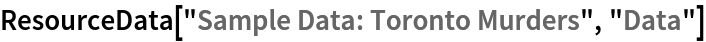Out=Summary of the spatial point data:

 In:=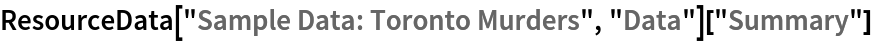Out=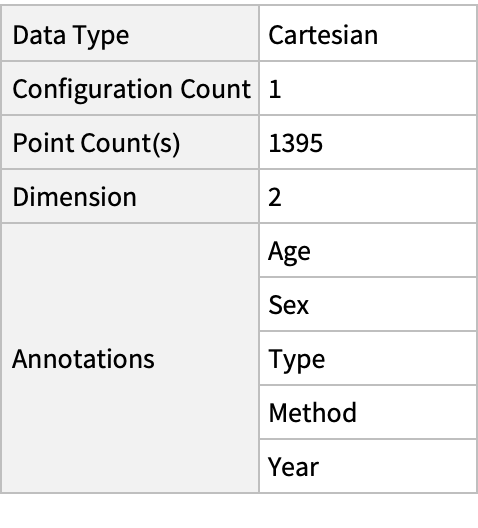### Visualizations (3)

Plot the spatial point data:

 In:=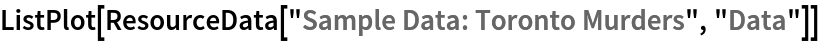Out=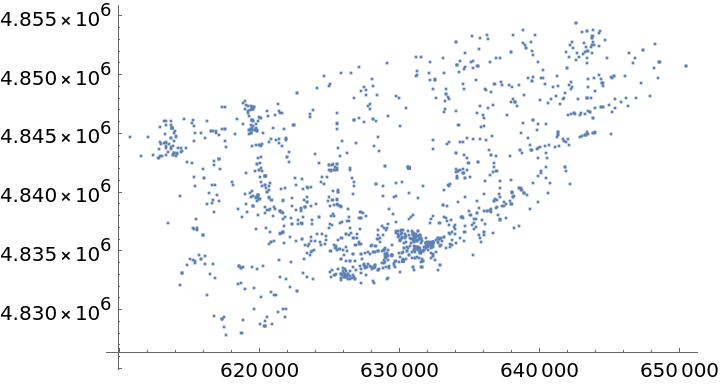Visualize the smooth point density:

 In:=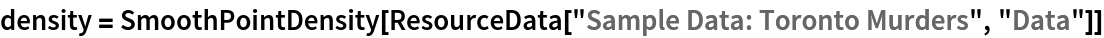Out=In:=Out=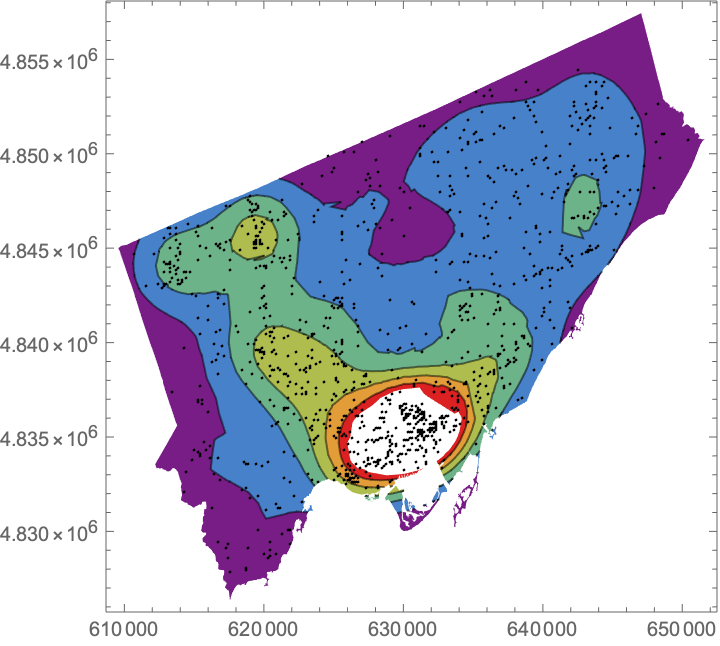Visualize the data with annotations:

 In:=Out=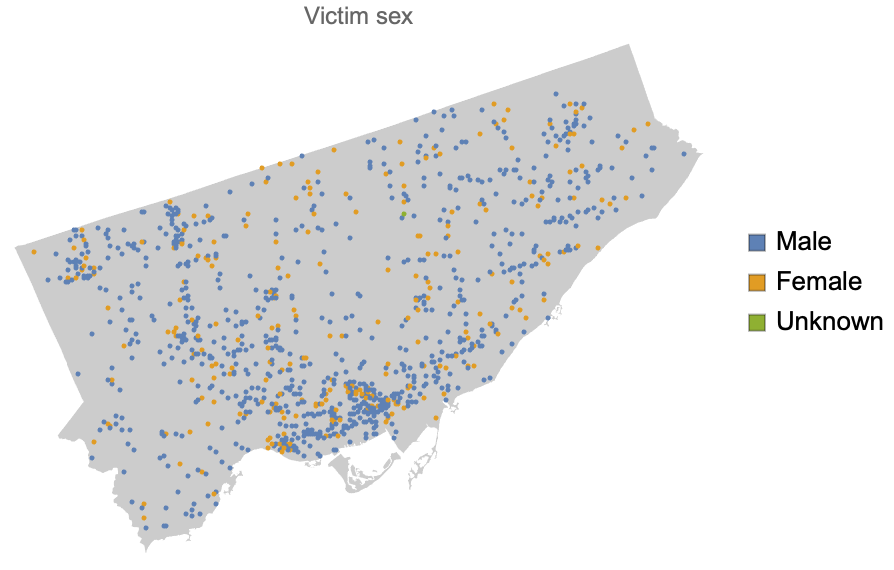### Analysis (2)

Compute probability of finding a point within given radius of an existing point - NearestNeighborG is the CDF of the nearest neighbor distribution:

 In:=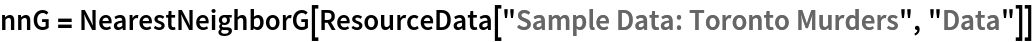Out=In:=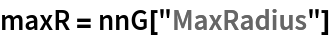Out=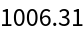In:=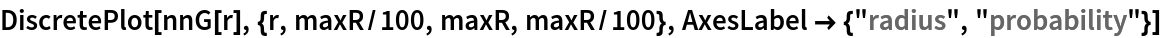Out=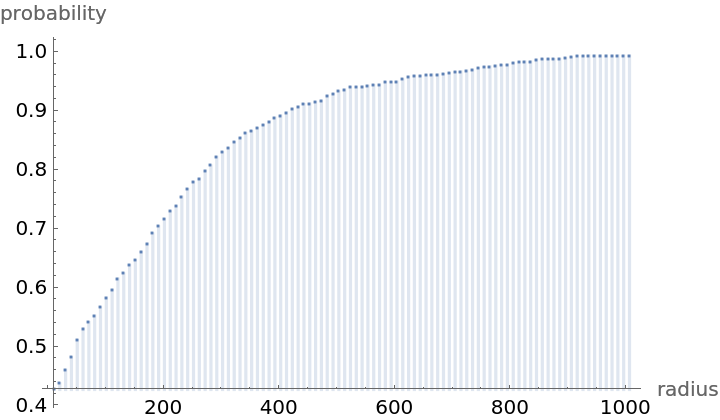Mean distance between a typical point and its nearest neighbor (for positive support distribution can be approximated via a Riemann sum of 1-CDF):

 In:=In:=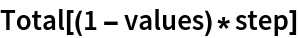Out=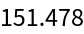In:=Out=Gosia Konwerska, "Sample Data: Toronto Murders" from the Wolfram Data Repository (2021)